# Properties of Kite

A closed figure made with 2 pairs of equal adjacent sides forms the shape of a kite.

In this mini-lesson, we will explore everything about kites.

You can observe the shape of a kite in the kites flown by kids in the sky.

So let's get started!

Do you see anything around that is Kite-shaped?## What Are the Properties of a Kite?

Let us quickly recall the shape Kite and its elements before we learn its properties.

### What Is a Kite?

A kite is a quadrilateral in which two pairs of adjacent sides are equal.

The elements of a kite are its 4 angles, its 4 sides, and 2 diagonals.

Let's look at the kite ABCD.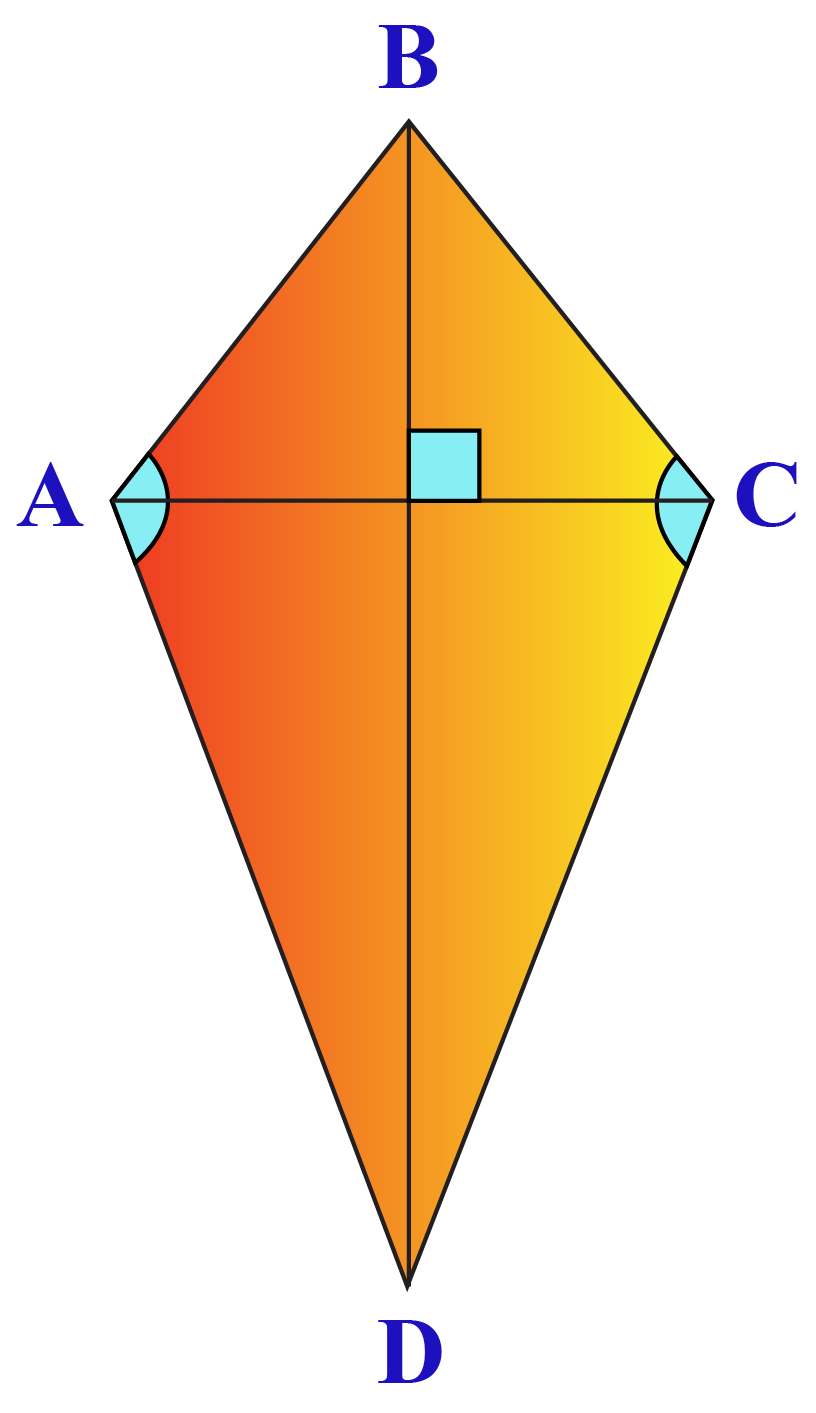The elements of this kite are:

• $$\angle ABC$$
• $$\angle BCD$$
• $$\angle CDA$$
• $$\angle DAB$$
• Side AB
• Side BC
• Side CD
• Diagonal AC
• Diagonal BD

### Properties of a Kite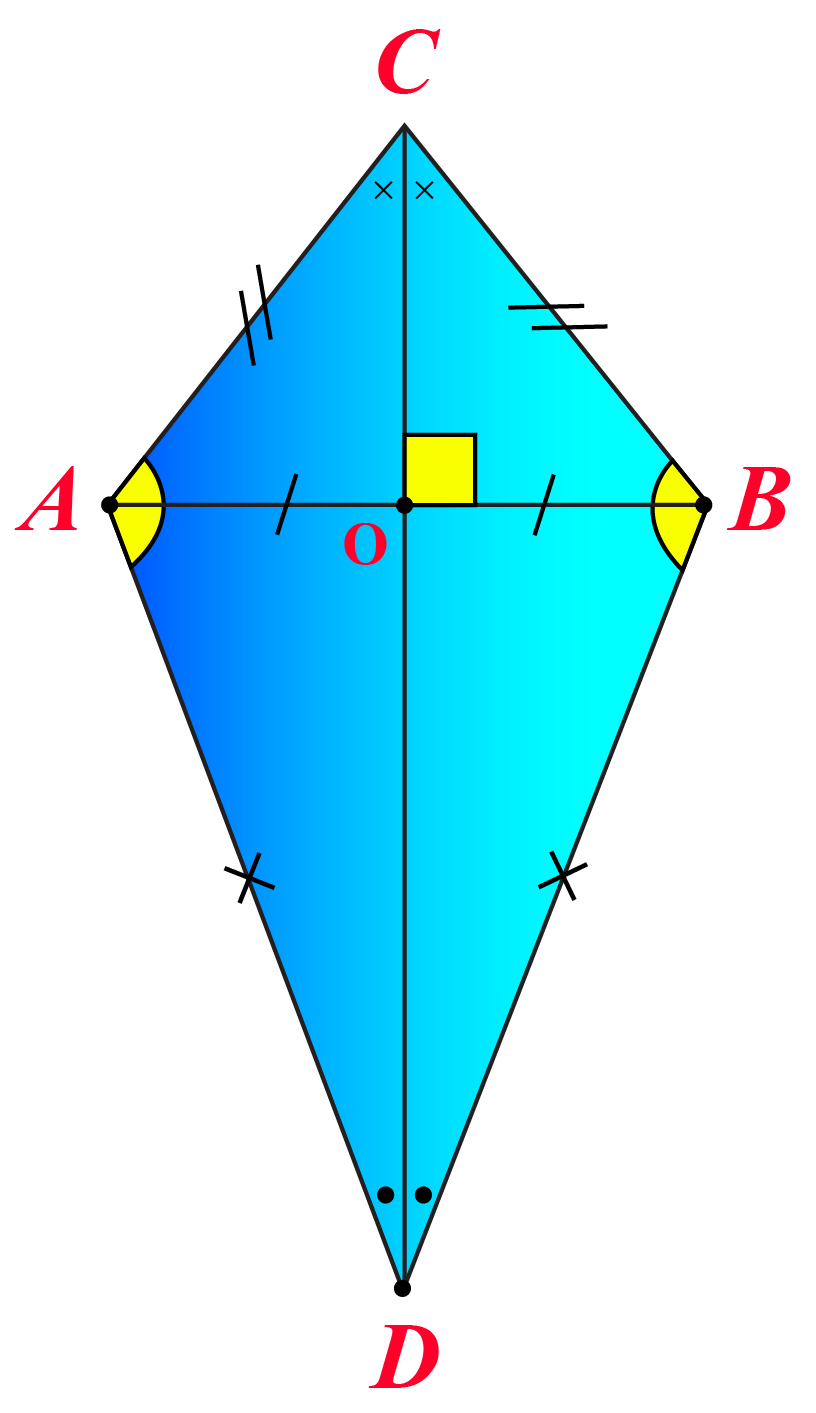A kite has:

• two pairs of adjacent equal sides

$$AC = BC$$ and $$AD = BD$$

• one pair of opposite angles (which is obtuse) that are equal

$$\angle A = \angle B$$

• diagonals that are perpendicular to each other

$$AB \perp CD$$

• a longer diagonal that bisects the shorter diagonal

$$AO=OB$$

• a longer diagonal that bisects the pair of opposite angles

$$\angle ACD = \angle DCB$$

$$\angle ADC = \angle CDB$$

Here is a simulation to understand the properties of a kite.

Drag any of the vertices in "red."

Observe how the angles and sides change while displaying the basic properties for that kite.

## How Do you Find the Area of a Kite?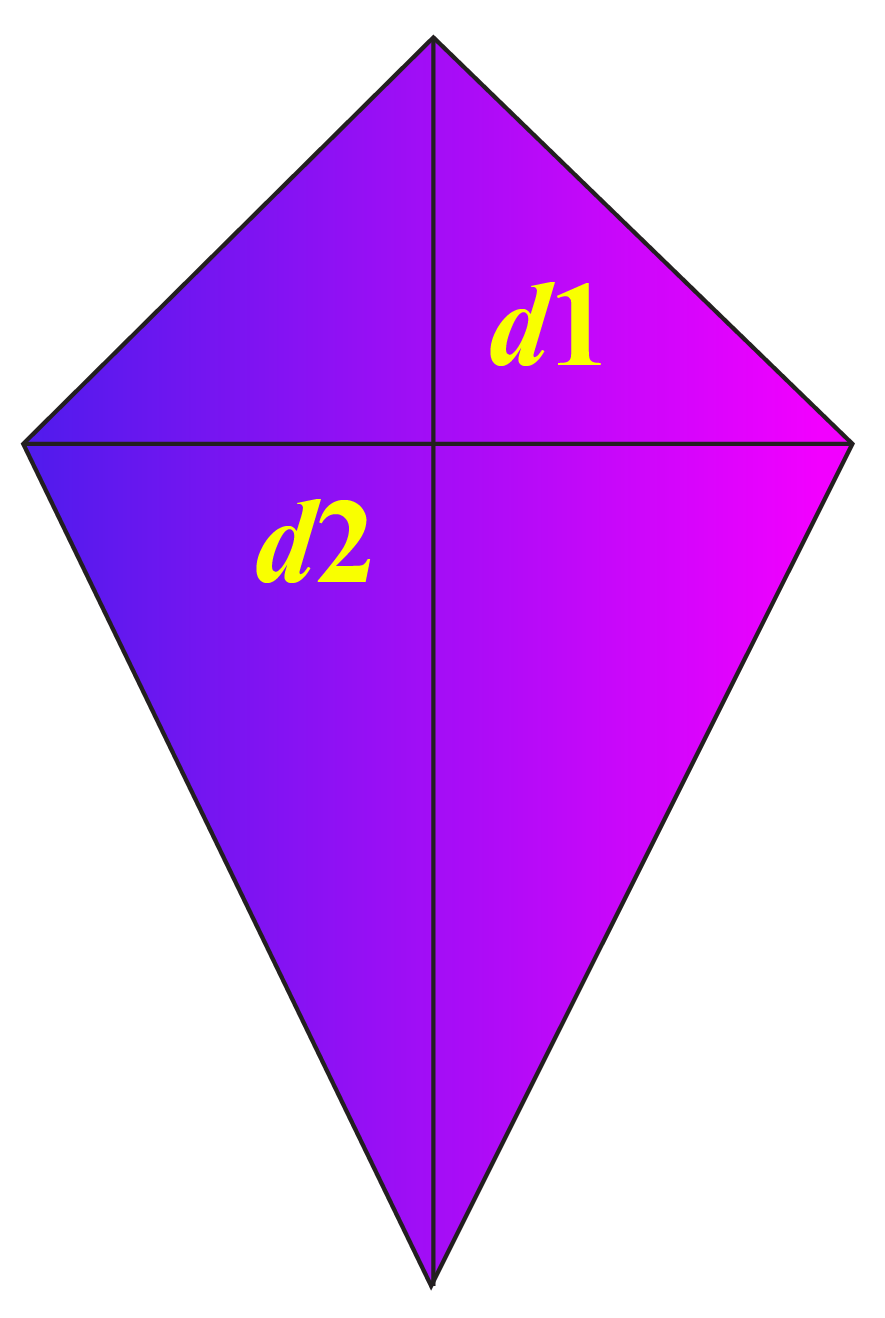The area of a kite is half the product of the lengths of its diagonals.

The formula to determine the area of a kite is:

 Area = $$\dfrac{1}{2}\times d_1 \times d_2$$Challenging Questions

 1 Can a kite be called a parallelogram? 2 Can a kite have sides 12 in, 25 in, 13 in, 25 in?

## Solved Examples

 Example 1

Brandon bought a kite whose diagonals are of length 10 in and 20 in.

He wants to find its area.

Can you help him?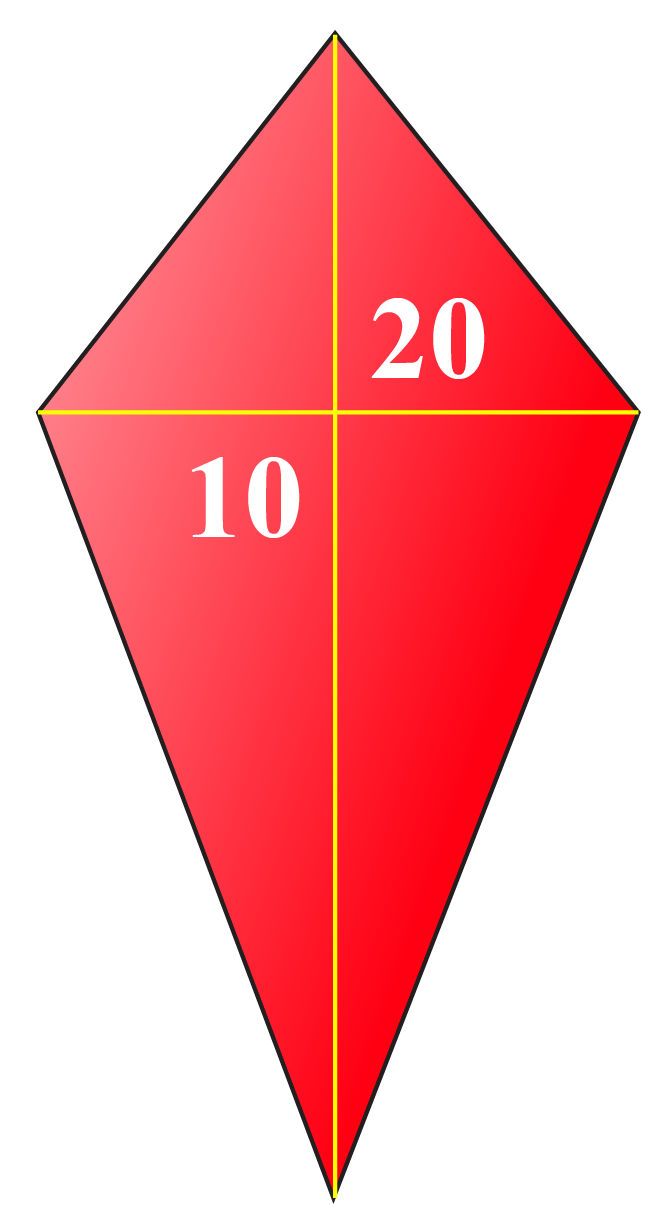Solution

The diagonals of the given kite are:

\begin{align} d_1&=10\text{ in}\\d_2&=20 \text{ in}\end{align}

The area of the kite is calculated using the formula:

\begin{align} A&=\dfrac{1}{2}\times d_1\times d_2\\&=\dfrac{1}{2}\times 10\times 20\\&=100 \text{ sq.in.}\end{align}

 $$\therefore$$ Area of the kite = $$100 \text{ in}^2$$
 Example 2

Four friends are flying kites in a park.

The lengths of the sides of each kite is 12 in, 12 in, 14 in, and 14 in

Determine the sum of the perimeters of the four kites.Solution

The sides of each kite are:

\begin{align} Side_1 &= 12 \text{ in}\\Side_2&=14 \text{ in}\end{align}

The perimeter of each kite is calculated using the formula:

\begin{align} P&=2\times (Side_1+Side_2)\\&=2\times (12+14)\\&=2\times 26\\&=52 \text{ in}\end{align}

The sum of the perimters of four kites is:

$$4\times 52=208 \text{ in}$$

 $$\therefore$$ The sum of the perimeters of the kites is 208 inches
 Example 3

Identify the pairs of equal sides in the given figure of kite below.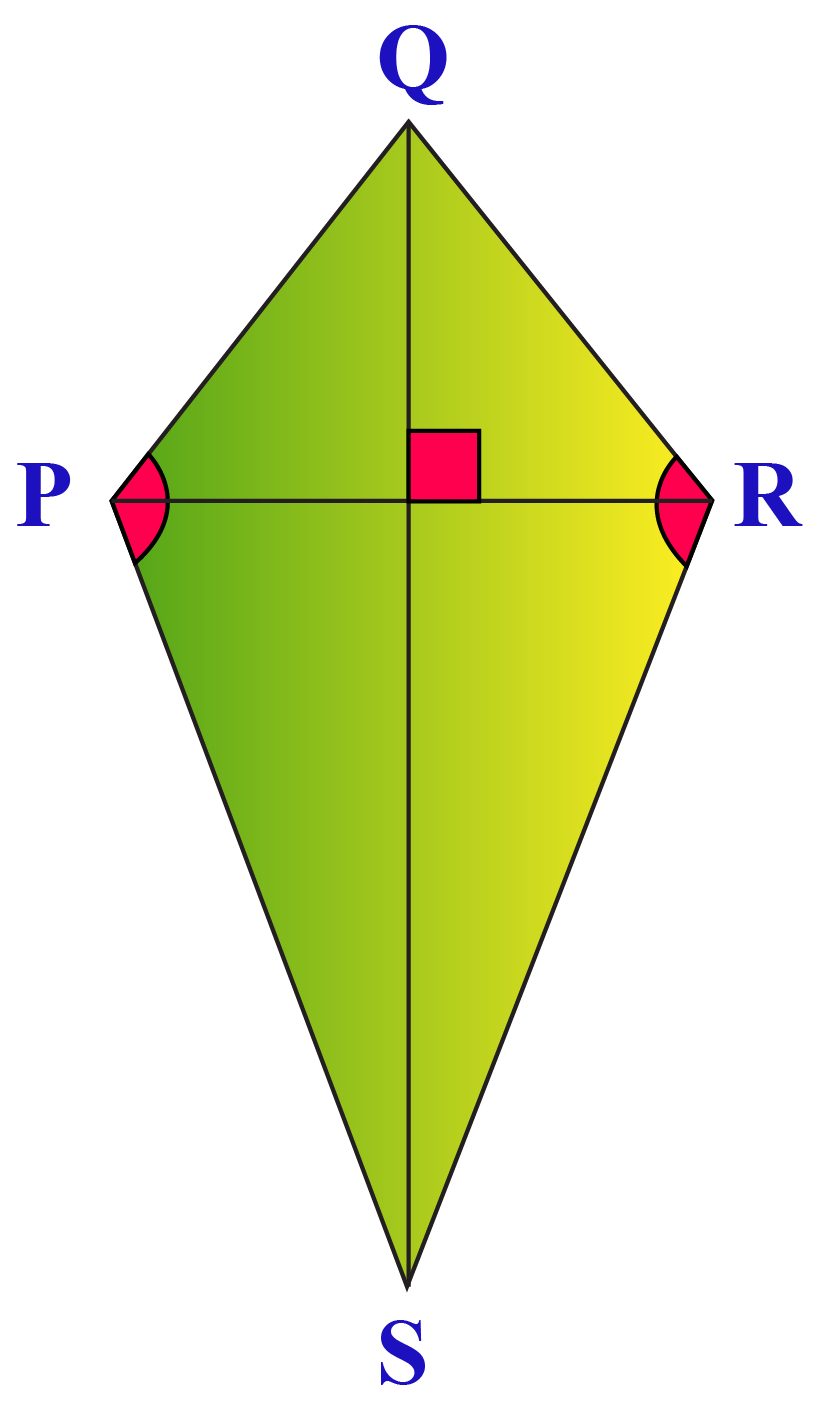Solution

We know that a kite has two pairs of equal adjacent sides.

The pairs of adjacent sides in the above kite are (PQ, QR), (PQ, PS), (QR, RS), and (PS, RS)

Pairs of equal adjacent sides are (PQ, QR) and (PS, RS)

 $$\therefore$$ Pairs of equal sides are (PQ, QR) and (PS, RS).

## Interactive Questions

Here are a few questions for you to practice.

## Let's Summarize

We hope you enjoyed learning about the Properties of a Kite with the simulations and practice questions. Now, you will be able to easily solve problems related to the properties of a kite.

At Cuemath, our team of math experts is dedicated to making learning fun for our favorite readers, the students!

Through an interactive and engaging learning-teaching-learning approach, the teachers explore all angles of a topic.

Be it worksheets, online classes, doubt sessions, or any other form of relation, it’s the logical thinking and smart learning approach that we at Cuemath believe in.

## 1. What is diagonal?

A diagonal is a line segment that joins the opposite vertices of a polygon.

## 2. Is a kite a parallelogram?

Opposite sides in a parallelogram are always parallel whereas this is not true for kites.Therefore, kite is not a parallelogram.

## 3. Can two angles of a kite be consecutive and supplementary?

No, two consecutive angles of a kite cannot be supplementary because if one pair of consecutive angles is supplementary, then another pair will also be supplementary.

Since a kite has one pair of equal opposite angles, therefore two pairs of opposite angles will have to be equal.

More Important Topics
Numbers
Algebra
Geometry
Measurement
Money
Data
Trigonometry
Calculus